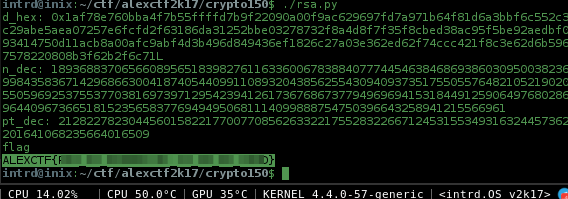# AlexCTF 2017 : crypto150-what_is_this_encryption

Fady assumed this time that you will be so n00b to tell what encryption he is using
he send the following note to his friend in plain sight:

``````p=0xa6055ec186de51800ddd6fcbf0192384ff42d707a55f57af4fcfb0d1dc7bd97055e8275cd4b78ec63c5d592f567c66393a061324aa2e6a8d8fc2a910cbee1ed9
q=0xfa0f9463ea0a93b929c099320d31c277e0b0dbc65b189ed76124f5a1218f5d91fd0102a4c8de11f28be5e4d0ae91ab319f4537e97ed74bc663e972a4a9119307
e=0x6d1fdab4ce3217b3fc32c9ed480a31d067fd57d93a9ab52b472dc393ab7852fbcb11abbebfd6aaae8032db1316dc22d3f7c3d631e24df13ef23d3b381a1c3e04abcc745d402ee3a031ac2718fae63b240837b4f657f29ca4702da9af22a3a019d68904a969ddb01bcf941df70af042f4fae5cbeb9c2151b324f387e525094c41
``````

He is underestimating our crypto skills!

## Solution

The encryption is RSA, and was given to us

• p = first prime number
• q = second prime number
• e = public/encryption exponent
• ct = ciphertext (encrypted text)

Quoted from wikipedia..

A user of RSA creates and then publishes a public key based on two large prime numbers, along with an auxiliary value. `The prime numbers must be kept secret`. Anyone can use the public key to encrypt a message, but with currently published methods, if the public key is large enough, only someone with knowledge of the prime numbers can feasibly decode the message.

Well, in this case we have the primes.. to decrypt we still need to find

• n = product of two primes (`p` and `q`)
• d = decryption key

### Extended Euclidean Algorithm

Ok, we have the two primes, `n` is easy to find, but how to recover decryption key?

We need to compute a polynomial greatest common divisor. To do this we used the Extended Euclidean algorithm, take a look at the articles if you like a lot of boring math stuff.

``````## Extended Euclidean Algorithm
def egcd(a, b):
x,y, u,v = 0,1, 1,0
while a != 0:
q, r = b//a, b%a
m, n = x-u*q, y-v*q
b,a, x,y, u,v = a,r, u,v, m,n
gcd = b
return gcd, x, y

n = p*q #product of primes
phi = (p-1)*(q-1) #modular multiplicative inverse
gcd, a, b = egcd(e, phi) #calling extended euclidean algorithm
d = a #a is decryption key
``````

Now pow `ciphered_text` `d` and `n` and we have the decrypted text.Flag: ALEXCTF{CENSURED}